# Uncertainty damping in kinetic traffic models by driver-assist controls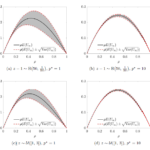Andrea Tosin, Mattia Zanella

Preprint arXiv, 2019.

In this paper, we propose a kinetic model of traffic flow with uncertain binary interactions, which explains the scattering of the fundamental diagram in terms of the macroscopic variability of aggregate quantities, such as the mean speed and the flux of the vehicles, produced by the microscopic uncertainty. Moreover, we design control strategies at the level of the microscopic interactions among the vehicles, by which we prove that it is possible to dampen the propagation of such an uncertainty across the scales. Our analytical and numerical results suggest that the aggregate traffic flow may be made more ordered, hence predictable, by implementing such control protocols in driver-assist vehicles. Remarkably, they also provide a precise relationship between a measure of the macroscopic damping of the uncertainty and the penetration rate of the driver-assist technology in the traffic stream.

|

# Monte Carlo gPC methods for diffusive kinetic flocking models with uncertainties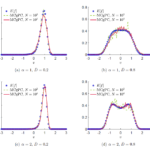José Antonio Carrillo, Mattia Zanella

Preprint arXiv, 2019.

In this paper we introduce and discuss numerical schemes for the approximation of kinetic equations for flocking behavior with phase transitions that incorporate uncertain quantities. This class of schemes here considered make use of a Monte Carlo approach in the phase space coupled with a stochastic Galerkin expansion in the random space. The proposed methods naturally preserve the positivity of the statistical moments of the solution and are capable to achieve high accuracy in the random space. Several tests on a kinetic alignment model with self propulsion validate the proposed methods both in the homogeneous and inhomogeneous setting, shading light on the influence of uncertainties in phase transition phenomena driven by noise such as their smoothing and confidence bands.

# Structure preserving stochastic Galerkin methods for Fokker-Planck equations with background interactions

Preprint arXiv, 2019.

This paper is devoted to the construction of structure preserving stochastic Galerkin schemes for Fokker-Planck type equations with uncertainties and interacting with an external distribution called the background. The proposed methods are capable to preserve physical properties in the approximation of statistical moments of the problem like nonnegativity, entropy dissipation and asymptotic behaviour of the expected solution. The introduced methods are second order accurate in the transient regimes and high order for large times. We present applications of the developed schemes to the case of fixed and dynamic background distribution for models of collective behaviour.

# Particle based gPC methods for mean-field models of swarming with uncertainty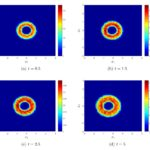José Antonio Carrillo, Lorenzo Pareschi, Mattia Zanella.

Communications in Computational Physics, 25(2): 508-531, 2019. (Preprint arXiv)

In this work we focus on the construction of numerical schemes for the approximation of stochastic mean–field equations which preserve the nonnegativity of the solution. The method here developed makes use of a mean-field Monte Carlo method in the physical variables combined with a generalized Polynomial Chaos (gPC) expansion in the random space. In contrast to a direct application of stochastic-Galerkin methods, which are highly accurate but lead to the loss of positivity, the proposed schemes are capable to achieve high accuracy in the random space without loosing nonnegativity of the solution. Several applications of the schemes to mean-field models of collective behavior are reported.

See more videos on my YouTube channel.

# Hybrid stochastic kinetic description of two-dimensional traffic dynamics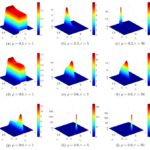Michael Herty, Andrea Tosin, Giuseppe Visconti, Mattia Zanella

SIAM Journal on Applied Mathematics, 78(5): 2737–2762, 2018. (Preprint arXiv)

In this work we present a two-dimensional kinetic traffic model which takes into account speed changes both when vehicles interact along the road lanes and when they change lane. Assuming that lane changes are less frequent than interactions along the same lane and considering that their mathematical description can be done up to some uncertainty in the model parameters, we derive a hybrid stochastic Fokker-Planck-Boltzmann equation in the quasi-invariant interaction limit. By means of suitable numerical methods, precisely structure preserving and direct Monte Carlo schemes, we use this equation to compute theoretical speed-density diagrams of traffic both along and across the lanes, including estimates of the data dispersion, and validate them against real data.

# Boltzmann-type models with uncertain binary interactions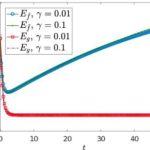Andrea Tosin, Mattia Zanella.

Communications in Mathematical Sciences, 16(4): 963-985, 2018. (Preprint arXiv)

In this paper we study binary interaction schemes with uncertain parameters for a general class of Boltzmann-type equations with applications in classical gas and aggregation dynamics. We consider deterministic (i.e., a priori averaged) and stochastic kinetic models, corresponding to different ways of understanding the role of uncertainty in the system dynamics, and compare some thermodynamic quantities of interest, such as the mean and the energy, which characterise the asymptotic trends. Furthermore, via suitable scaling techniques we derive the corresponding deterministic and stochastic Fokker-Planck equations in order to gain more detailed insights into the respective asymptotic distributions. We also provide numerical evidences of the trends estimated theoretically by resorting to recently introduced structure preserving uncertainty quantification methods.

# Uncertainty quantification for kinetic models in socio-economic and life sciences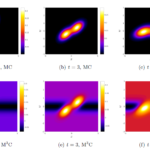Giacomo Dimarco, Lorenzo Pareschi, Mattia Zanella

Uncertainty Quantification for Hyperbolic and Kinetic Equations, Eds. S. Jin, L. Pareschi, SEMA-SIMAI Springer Series vol.14, Springer-Cham, 2017. (Preprint arXiv)

Kinetic equations play a major rule in modeling large systems of interacting particles. Recently the legacy of classical kinetic theory found novel applications in socio-economic and life sciences, where processes characterized by large groups of agents exhibit spontaneous emergence of social structures. Well-known examples are the formation of clusters in opinion dynamics, the appearance of inequalities in wealth distributions, flocking and milling behaviors in swarming models, synchronization phenomena in biological systems and lane formation in pedestrian traffic. The construction of kinetic models describing the above processes, however, has to face the difficulty of the lack of fundamental principles since physical forces are replaced by empirical social forces. These empirical forces are typically constructed with the aim to reproduce qualitatively the observed system behaviors, like the emergence of social structures, and are at best known in terms of statistical information of the modeling parameters. For this reason the presence of random inputs characterizing the parameters uncertainty should be considered as an essential feature in the modeling process. In this survey we introduce several examples of such kinetic models, that are mathematically described by nonlinear Vlasov and Fokker–Planck equations, and present different numerical approaches for uncertainty quantification which preserve the main features of the kinetic solution.

# Uncertainty Quantification in Control Problems for Flocking Models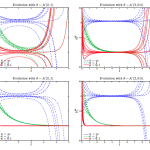Giacomo Albi, Lorenzo Pareschi, Mattia Zanella

Mathematical Problems in Engineering, Vol. 2015.  (Preprint arXiv)

In this paper the optimal control of flocking models with random inputs is investigated from a numerical point of view. The effect of uncertainty in the interaction parameters is studied for a Cucker-Smale type model using a generalized polynomial chaos (gPC) approach. Numerical evidence of threshold effects in the alignment dynamic due to the random parameters is given. The use of a selective model predictive control permits to steer the system towards the desired state even in unstable regimes.

|